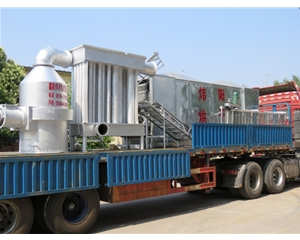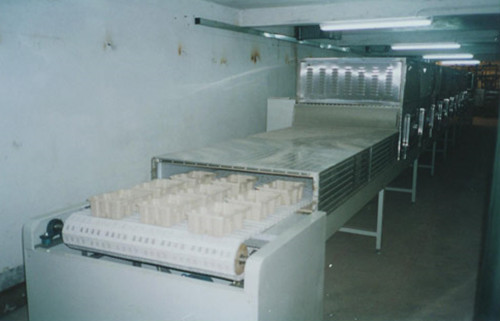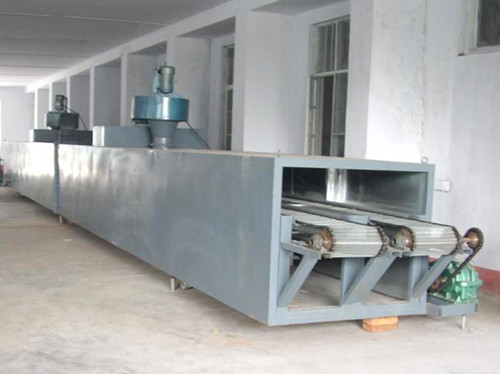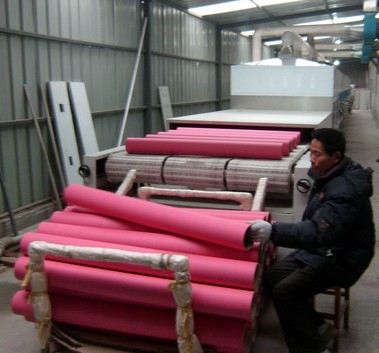`型号` `DW-1.2-8` `DW-1.2-10` `DW-1.6-8` `DW-1.6-10` `DW-2-8` `DW-2-10` `单元数` `4` `5` `4` `5` `4` `5` `带宽 (m)` `1.2` `1.6` `2` `干燥段长 (m)` `8` `10` `8` `10` `8` `10` `铺料厚(mm)` `10-80` `使用温度 (℃）` `50-120` `蒸汽压力 (Mpa)` `0.2-0.8` `蒸汽耗量 (kg/h)` `120-130` `150-375` `150-375` `170-470` `180-500` `225-600` `换热面积 (m^2)` `272` `340` `352` `440` `448` `560` `干燥时间 (h)` `0.2-1.2` `0.25-1.5` `0.2-1.2` `0.25-1.5` `0.2-1.2` `0.25-1.5` `干燥强度 (kg)水/h` `60-160` `80-220` `75-220` `95-250` `100-260` `120-300` `风机数量` `5` `6` `5` `6` `5` `6` `风机总功率(kw)` `6.6` `8.8` `9` `12` `12` `16` `设备总功率 (kw)` `11.4` `13.6` `11.4` `13.6` `19.7` `23.7` `外形尺寸` `长 (m)` `9.77` `11.77` `9.77` `11.77` `9.77` `11.77` `宽 (m)` `1.5` `1.5` `2.24` `2.24` `2.66` `2.66` `高 (m)` `2.83` `2.83` `2.83` `2.83` `3.50` `3.50` `总重 (kg)` `4800` `5780` `5400` `6550` `6350` `7800` ``` ```

#### 关注我们